# NCERT Exemplar Class 9 Science Solutions for Chapter 11 - Work and Energy

## NCERT Exemplar Solutions Class 9 Science Chapter 11 – Free PDF Download

NCERT Exemplar Solutions for Class 9 Science Chapter 11 Work and Energy provided here will help you in practising a range of questions effectively. This NCERT Exemplar will boost your confidence by helping you in understanding the concepts from the exam point of view. Work and energy are important concepts of Physics. Having complete knowledge of the terms related to work and energy is imperative to learn the higher-level concepts of Physics in higher classes. To master the concepts of work and energy, solve NCERT Exemplar Class 9 Science Chapter 11 Work and Energy.

Studying this Exemplar is crucial, as it presents you with work and energy Class 9 MCQs, numerical problems, HOTS and short answer questions. There are 9 MCQs, 11 short answer questions and 8 long answer questions to boost your exam preparation. Visit the below links to get the NCERT Exemplar for Class 9 Science Chapter 11.

### Download the PDF of NCERT Exemplar for Class 9 Science Chapter 11 – Work and Energy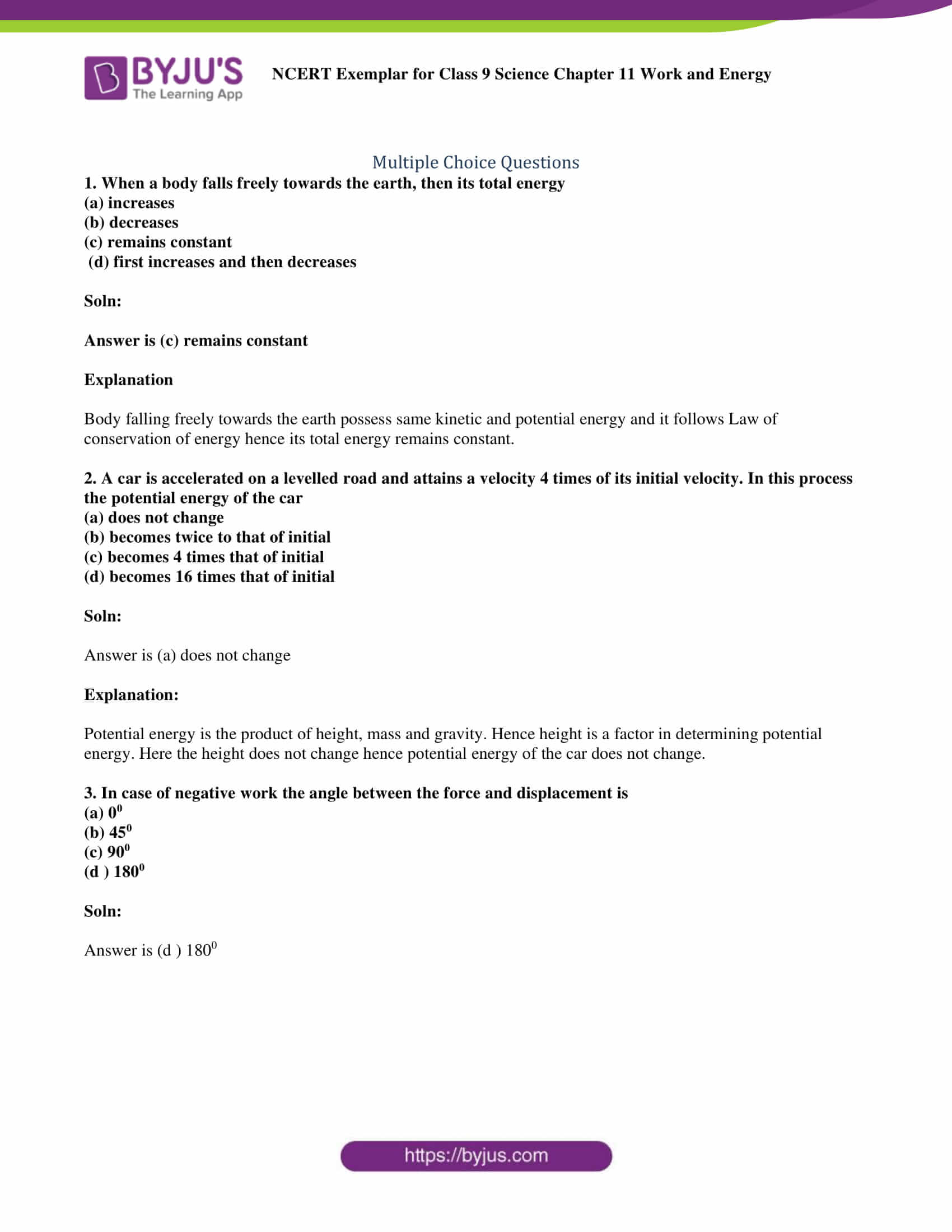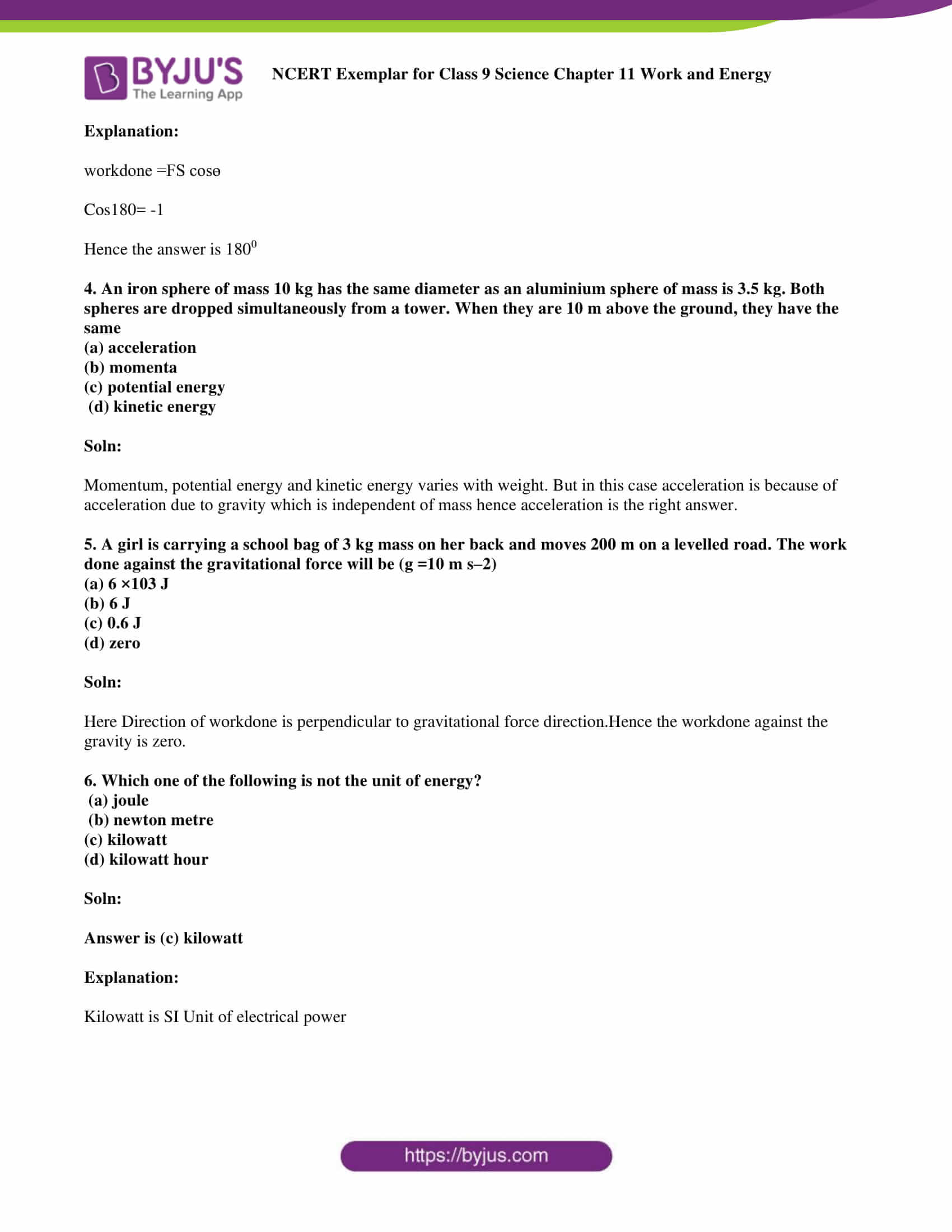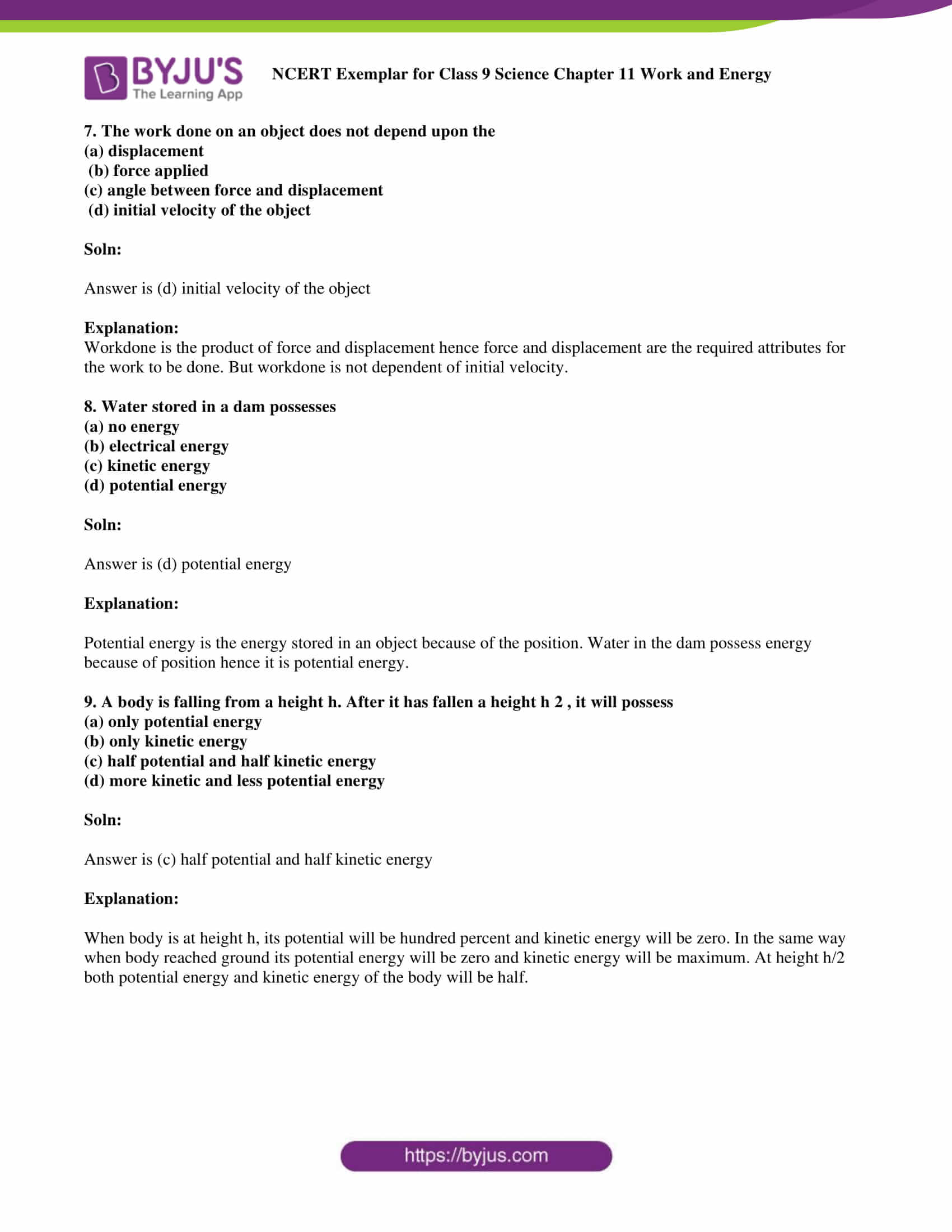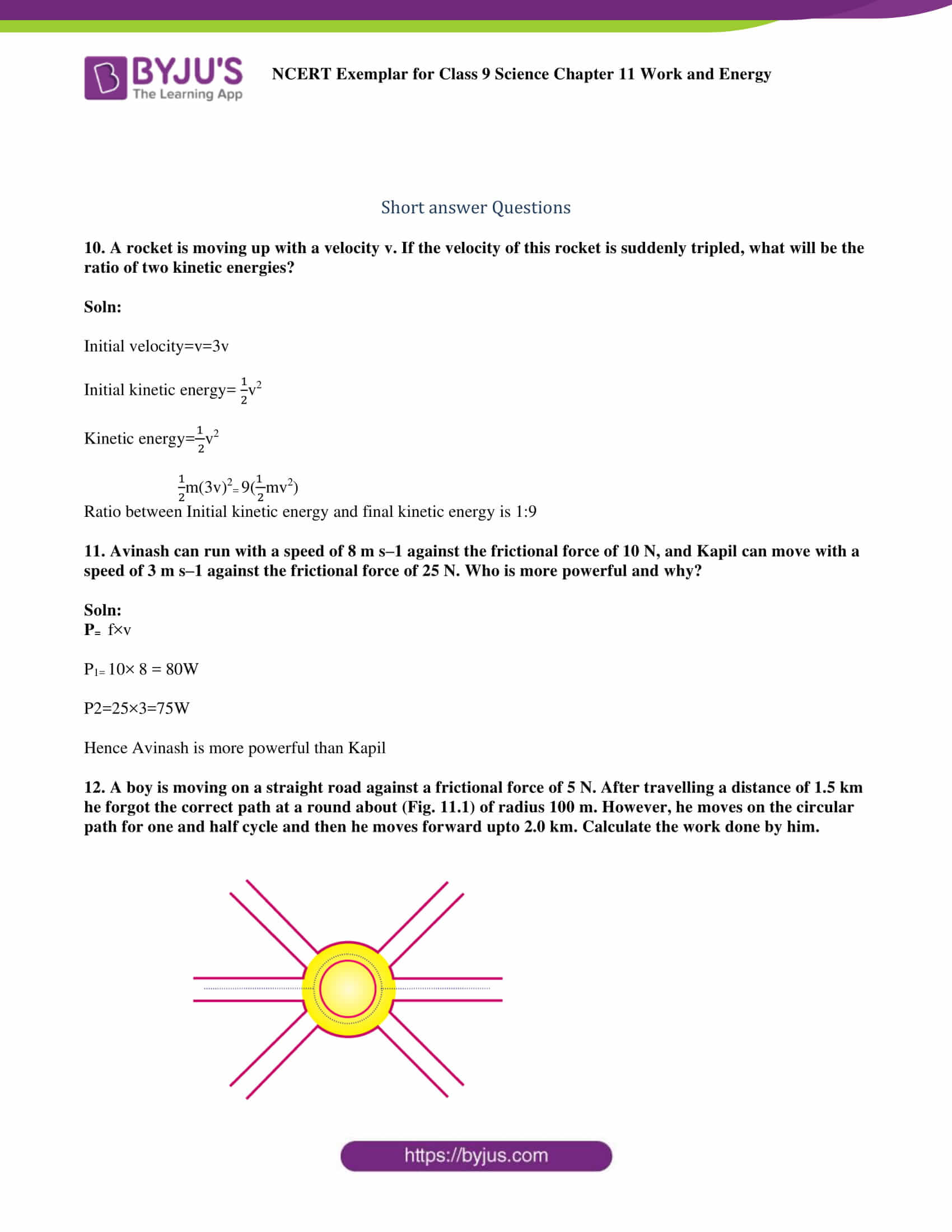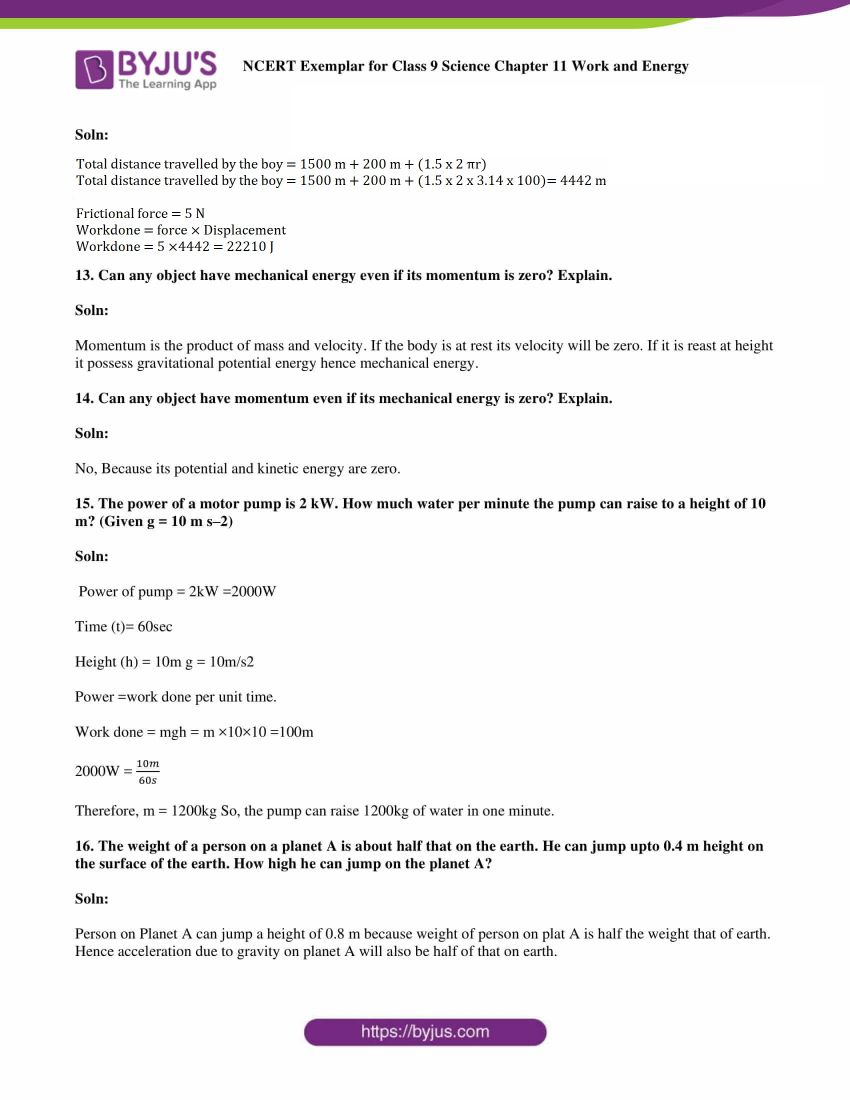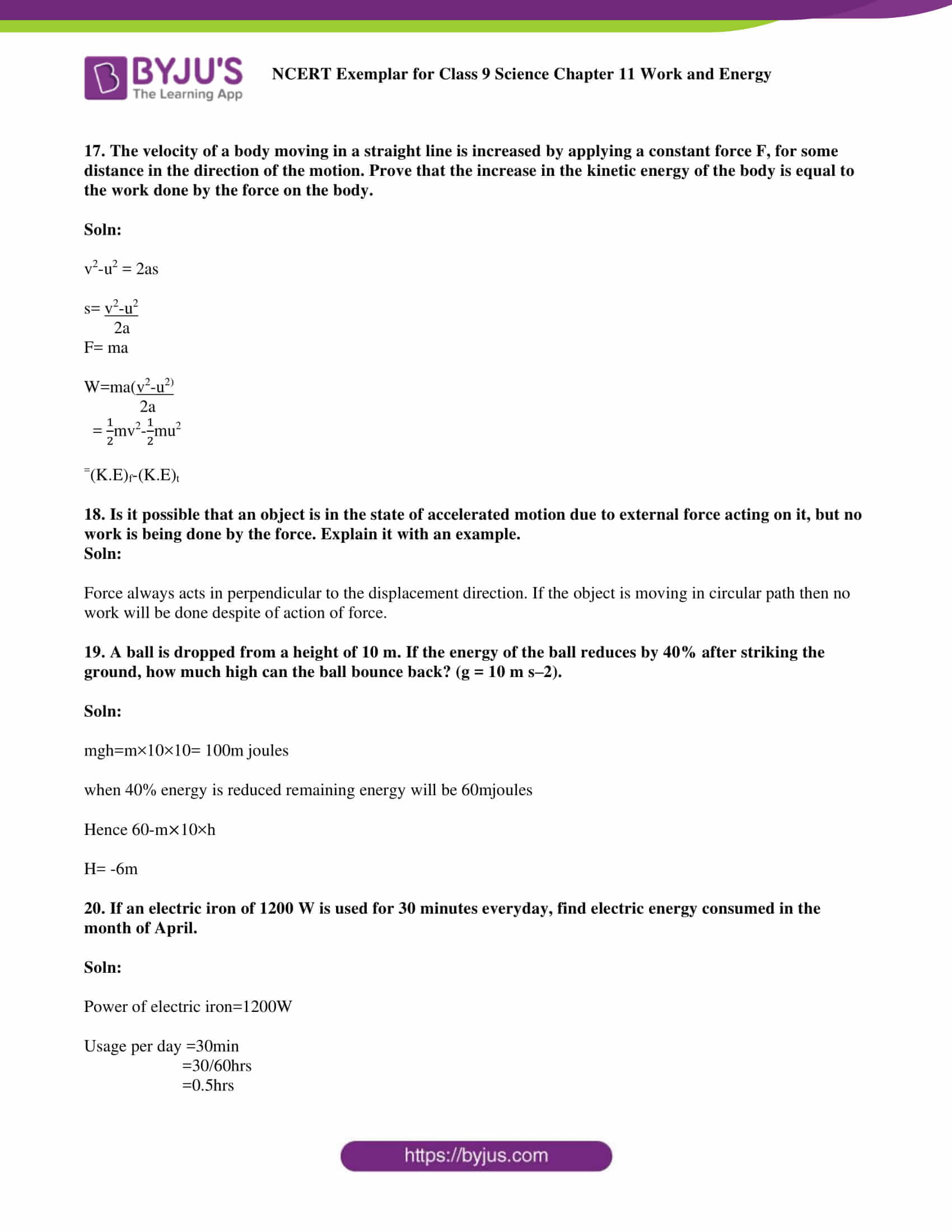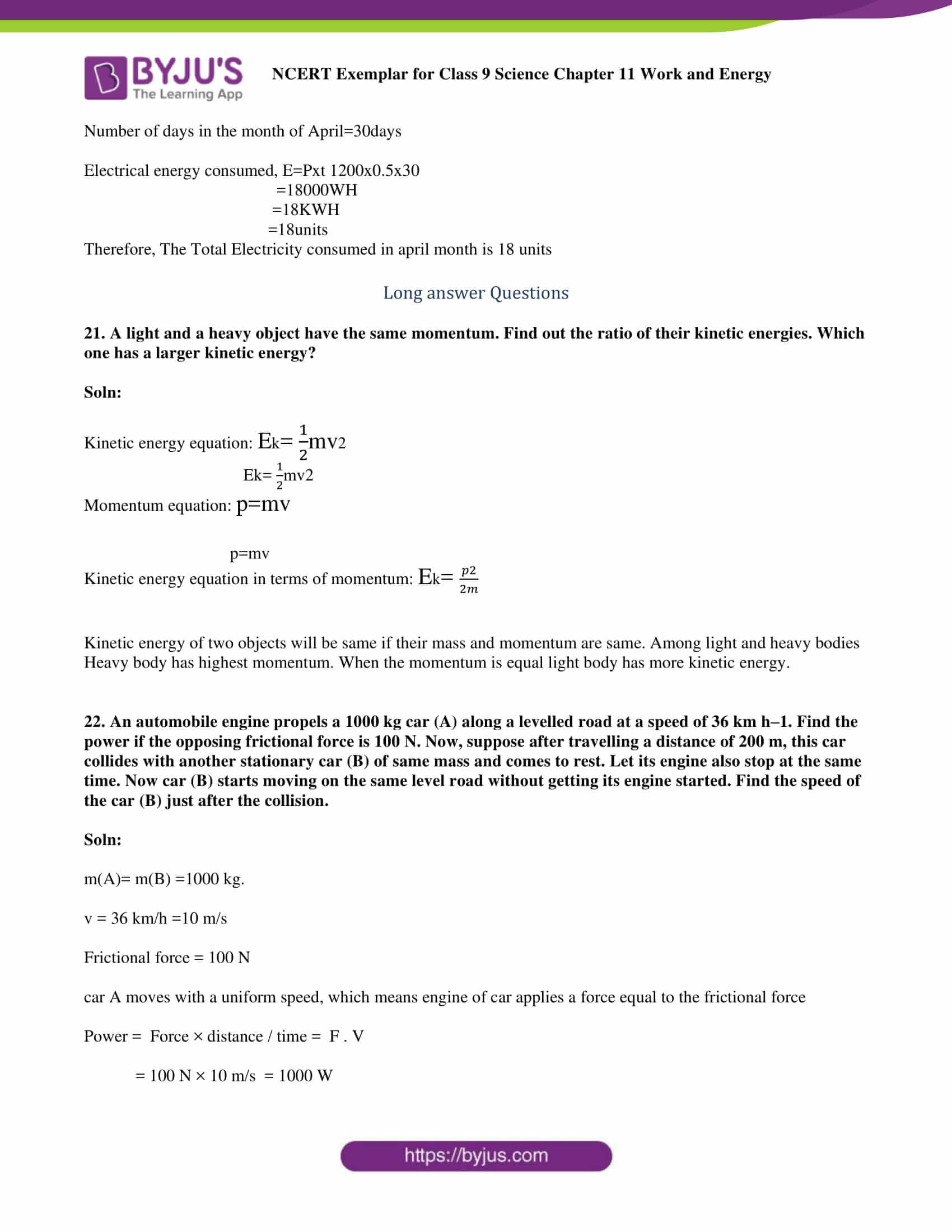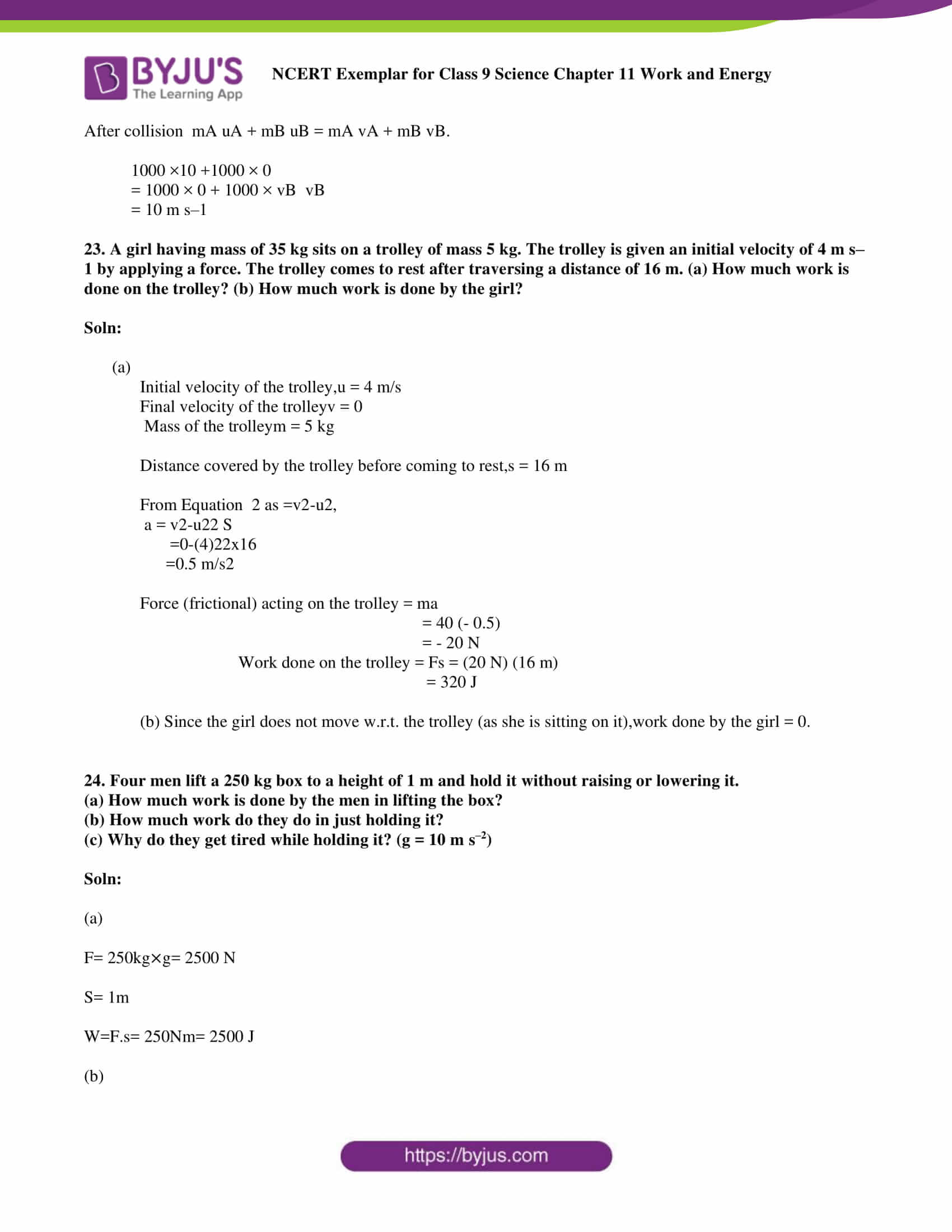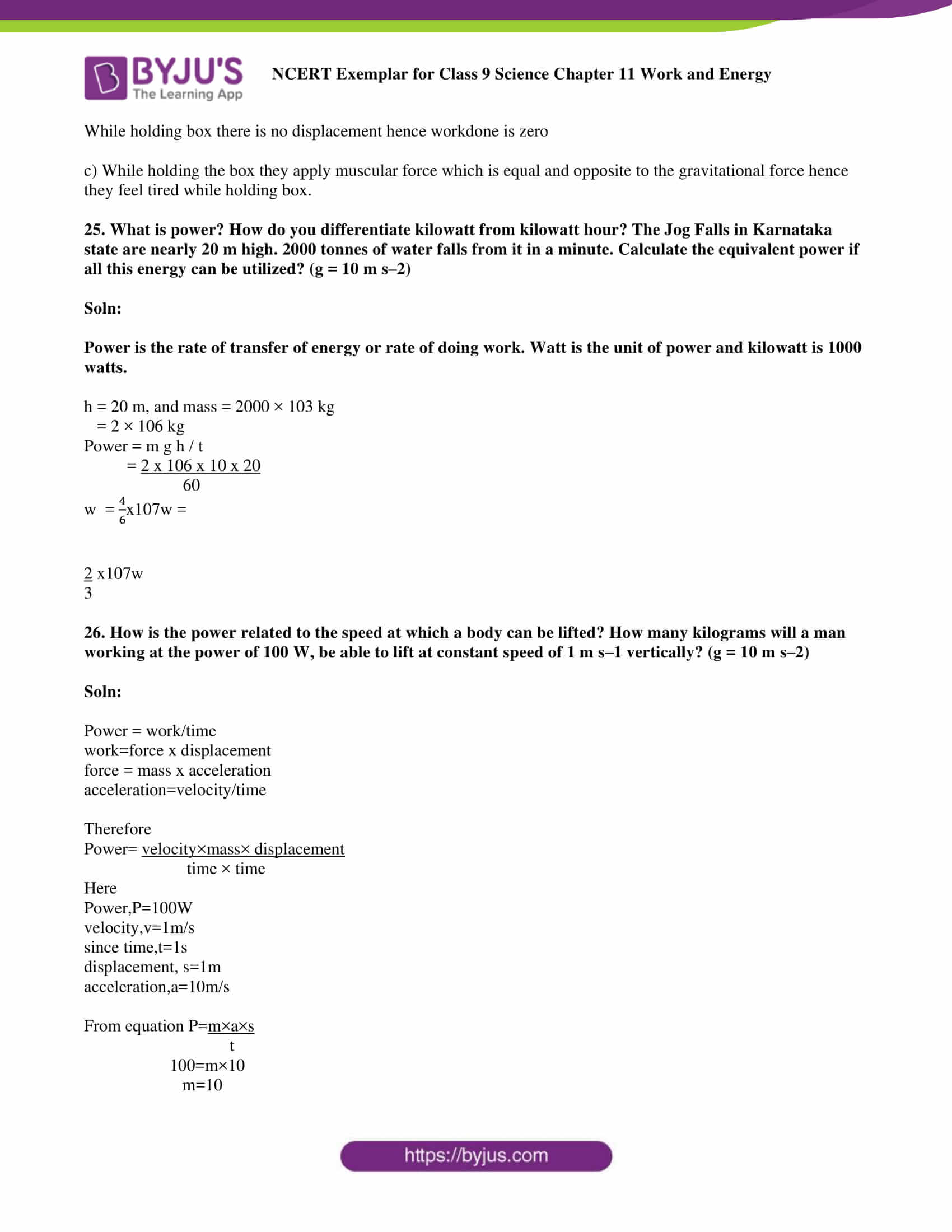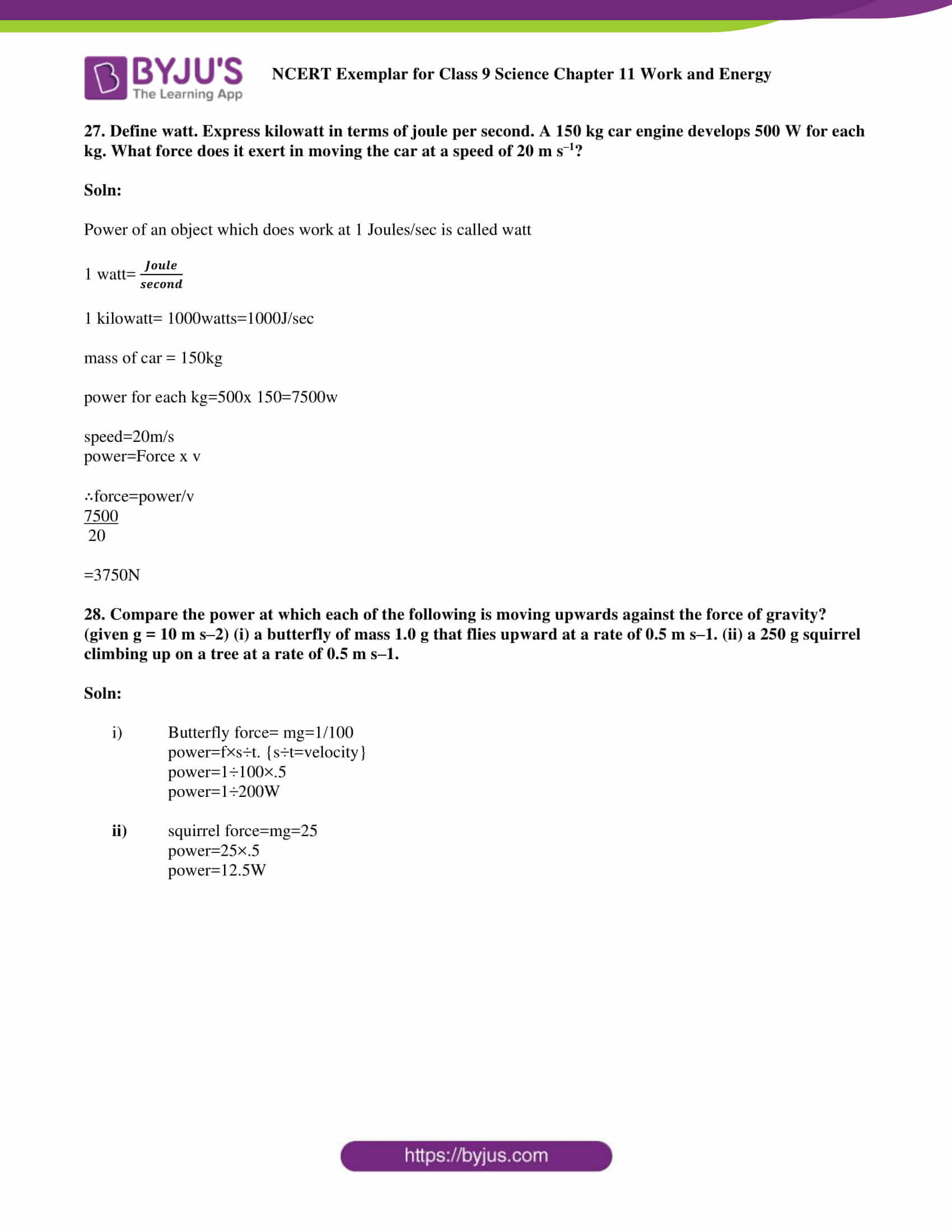### Multiple Choice Questions

1. When a body falls freely towards the earth, then its total energy

(a) increases

(b) decreases

(c) remains constant

(d) first increases and then decreases

Soln:

Explanation

Body falling freely towards the earth possess the same kinetic and potential energy and it follows the Law of conservation of energy hence its total energy remains constant.

2. A car is accelerated on a levelled road and attains a velocity 4 times of its initial velocity. In this process the potential energy of the car

(a) does not change

(b) becomes twice to that of initial

(c) becomes 4 times that of initial

(d) becomes 16 times that of initial

Soln:

Answer is (a) does not change

Explanation:

Potential energy is the product of height, mass and gravity. Hence height is a factor in determining potential energy. Here the height does not change hence potential energy of the car does not change.

3. In case of negative work the angle between the force and displacement is

(a) 00

(b) 450

(c) 900

(d ) 1800

Soln:

Explanation:

work done = FS cosɵ

Cos180 = -1

4. An iron sphere of mass 10 kg has the same diameter as an aluminium sphere of mass is 3.5 kg. Both spheres are dropped simultaneously from a tower. When they are 10 m above the ground, they have the same

(a) acceleration

(b) momenta

(c) potential energy

(d) kinetic energy

Soln:

(a) acceleration

Explanation:

Momentum, potential energy and kinetic energy varies with weight. But in this case, acceleration is because of acceleration due to gravity which is independent of mass hence acceleration is the right answer.

5. A girl is carrying a school bag of 3 kg mass on her back and moves 200 m on a levelled road. The work done against the gravitational force will be (g =10 m s–2)

(a) 6 ×103 J

(b) 6 J

(c) 0.6 J

(d) zero

Soln:

(d) zero

Explanation:

Here Direction of work done is perpendicular to gravitational force direction. Hence the work done against the gravity is zero.

6. Which one of the following is not the unit of energy?

(a) joule

(b) newton metre

(c) kilowatt

(d) kilowatt-hour

Soln:

Explanation:

Kilowatt is the SI Unit of electrical power

7. The work done on an object does not depend upon the

(a) displacement

(b) force applied

(c) angle between force and displacement

(d) initial velocity of the object

Soln:

Answer is (d) initial velocity of the object

Explanation:

Work done is the product of force and displacement hence force and displacement are the required attributes for the work to be done. But work done is not dependent on initial velocity.

8. Water stored in a dam possesses

(a) no energy

(b) electrical energy

(c) kinetic energy

(d) potential energy

Soln:

Explanation:

Potential energy is the energy stored in an object because of the position. Water in the dam possesses energy because of position hence it is potential energy.

9. A body is falling from a height h. After it has fallen from a height h2, it will possess

(a) only potential energy

(b) only kinetic energy

(c) half potential and half kinetic energy

(d) more kinetic and less potential energy

Soln:

Answer is (c) half potential and half kinetic energy

Explanation:

When body is at height h, its potential will be hundred per cent and kinetic energy will be zero. In the same way, when the body reaches the ground its potential energy will be zero and kinetic energy will be maximum. At height h/2 both potential energy and kinetic energy of the body will be half.

10. A rocket is moving up with a velocity v. If the velocity of this rocket is suddenly tripled, what will be the ratio of two kinetic energies?

Soln:

Initial velocity = v = 3v

Initial kinetic energy =

$$\begin{array}{l}\frac{1}{2}\end{array}$$
v2

Kinetic energy =

$$\begin{array}{l}\frac{1}{2}\end{array}$$
v2

$$\begin{array}{l}\frac{1}{2}\end{array}$$
m(3v)2 = 9(
$$\begin{array}{l}\frac{1}{2}\end{array}$$
mv2)

Ratio between Initial kinetic energy and final kinetic energy is 1:9

11. Avinash can run with a speed of 8 m s–1 against the frictional force of 10 N, and Kapil can move with a speed of 3 m s–1 against the frictional force of 25 N. Who is more powerful and why?

Soln:

P= f×v

P1= 10× 8 = 80W

P2=25×3=75W

Hence Avinash is more powerful than Kapil

12. A boy is moving on a straight road against a frictional force of 5 N. After travelling a distance of 1.5 km he forgot the correct path at a round about (Fig. 11.1) of radius 100 m. However, he moves on the circular path for one and half cycle and then he moves forward upto 2.0 km. Calculate the work done by him.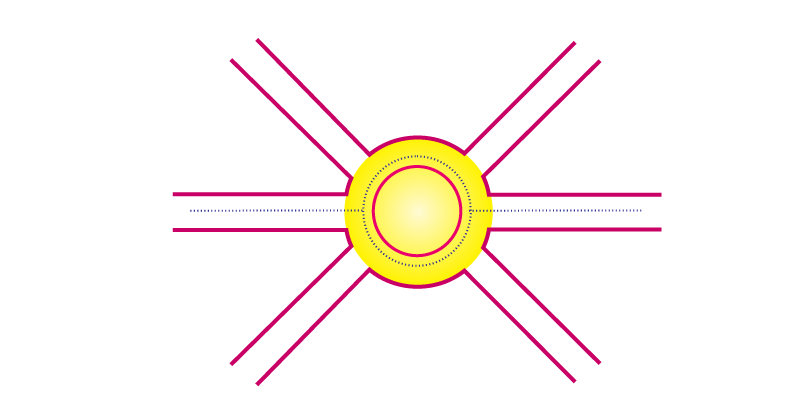Soln:

Total distance travelled by the boy = 1500 m + 200 m + (1.5 x 2 πr)

Total distance travelled by the boy = 1500 m + 200 m + (1.5 x 2 x 3.14 x 100)= 4442 m

Frictional force = 5 N

Workdone = force × Displacement

Workdone = 5 ×4442 = 22210 J

13. Can any object have mechanical energy even if its momentum is zero? Explain.

Soln:

Momentum is the product of mass and velocity. If the body is at rest its velocity will be zero. If it is rest at the height it possesses gravitational potential energy hence mechanical energy.

14. Can any object have momentum even if its mechanical energy is zero? Explain.

Soln:

No, Because its potential and kinetic energy are zero.

15. The power of a motor pump is 2 kW. How much water per minute the pump can raise to a height of 10 m? (Given g = 10 m s–2)

Soln:

Power of pump = 2kW =2000W

Time (t)= 60sec

Height (h) = 10m g = 10m/s2

Power =work done per unit time.

Work done = mgh = m ×10×10 =100m

= 2000W

Therefore, m = 1200 kg So, the pump can raise 1200kg of water in one minute.

16. The weight of a person on a planet A is about half that on the earth. He can jump upto 0.4 m height on the surface of the earth. How high he can jump on the planet A?

Soln:

Person on planet A can jump a height of 0.8 m because the weight of the person on plat A is half the weight that of earth. Hence acceleration due to gravity on planet A will also be half of that on earth.

17. The velocity of a body moving in a straight line is increased by applying a constant force F, for some distance in the direction of the motion. Prove that the increase in the kinetic energy of the body is equal to the work done by the force on the body.

Soln:

v2-u2 = 2as

s= v2-u2/2a

F = ma

W = ma(v2-u2)/2a

=

$$\begin{array}{l}\frac{1}{2}\end{array}$$
mv2 -
$$\begin{array}{l}\frac{1}{2}\end{array}$$
mu2

=(K.E)f-(K.E)t

18. Is it possible that an object is in the state of accelerated motion due to external force acting on it, but no work is being done by the force? Explain it with an example.

Soln:

Force always acts in perpendicular to the displacement direction. If the object is moving in circular path then no work will be done despite of action of a force.

19. A ball is dropped from a height of 10 m. If the energy of the ball reduces by 40% after striking the ground, how much high can the ball bounce back? (g = 10 m s–2).

Soln:

mgh=m×10×10= 100m joules

when 40% energy is reduced remaining energy will be 60mjoules

Hence 60 – m x 10 × h

H = -6m

20. If an electric iron of 1200 W is used for 30 minutes everyday, find electric energy consumed in the month of April.

Soln:

Power of electric iron=1200W

Usage per day = 30min

=30/60hrs

=0.5hrs

Number of days in the month of April=30days

Electrical energy consumed, E=Pxt 1200×0.5×30

=18000WH

=18KWH

=18units

Therefore, The Total Electricity consumed in April month is 18 units

21. A light and a heavy object have the same momentum. Find out the ratio of their kinetic energies. Which one has a larger kinetic energy?

Soln:

Kinetic energy equation: Ek=1/2 mv2

Momentum equation: p=mv

Kinetic energy equation in terms of momentum: Ek = p2/2m

Kinetic energy of two objects will be the same if their mass and momentum are the same. Among light and heavy bodies, Heavy body has highest momentum. When the momentum is the equal light body has more kinetic energy.

22. An automobile engine propels a 1000 kg car (A) along a levelled road at a speed of 36 km h–1. Find the power if the opposing frictional force is 100 N. Now, suppose after travelling a distance of 200 m, this car collides with another stationary car (B) of same mass and comes to rest. Let its engine also stop at the same time. Now car (B) starts moving on the same level road without getting its engine started. Find the speed of the car (B) just after the collision.

Soln:

m(A)= m(B) = 1000 kg.

v = 36 km/h =10 m/s

Frictional force = 100 N

car A moves with a uniform speed, which means engine of car applies a force equal to the frictional force

Power =  Force × distance / time =  F . V

= 100 N × 10 m/s  = 1000 W

After collision  mA uA + mB uB = mA vA + mB vB.

1000 × 10 + 1000 × 0

= 1000 × 0 + 1000 × vB  vB

= 10 m s–1

23. A girl having a mass of 35 kg sits on a trolley of mass 5 kg. The trolley is given an initial velocity of 4 m s–1 by applying a force. The trolley comes to rest after traversing a distance of 16 m. (a) How much work is done on the trolley? (b) How much work is done by the girl?

Soln:

Initial velocity of the trolley,u = 4 m/s

Final velocity of the trolley v = 0

Mass of the trolley m = 5 kg

Distance covered by the trolley before coming to rest,s = 16 m

From Equation 2 as = v2-u2,

a = v2-u22 S

= 0-(4)22×16

= 0.5 m/s2

Force (frictional) acting on the trolley = ma

= 40 (- 0.5)

= – 20 N

Work done on the trolley = Fs = (20 N) (16 m)

= 320 J

(b) Since the girl does not move w.r.t. the trolley (as she is sitting on it), work done by the girl = 0.

24. Four men lift a 250 kg box to a height of 1 m and hold it without raising or lowering it.

(a) How much work is done by the men in lifting the box?

(b) How much work do they do in just holding it?

(c) Why do they get tired while holding it? (g = 10 m s–2)

Soln:

(a)

F= 250kg x g = 2500 N

S= 1m

W=F.s= 250Nm = 2500 J

(b)

While holding box there is no displacement hence work done is zero

c) While holding the box they apply a muscular force which is equal and opposite to the gravitational force hence they feel tired while holding box.

25. What is power? How do you differentiate kilowatt from kilowatt-hour? The Jog Falls in Karnataka state are nearly 20 m high. 2000 tonnes of waterfalls from it in a minute. Calculate the equivalent power if all this energy can be utilized? (g = 10 m s–2)

Soln:

Power is the rate of transfer of energy or the rate of doing work. Watt is the unit of power and kilowatt is 1000 watts.

h = 20 m, and mass = 2000 × 103 kg

= 2 × 106 kg

Power = m g h / t

= 2 x 106 x 10 x 20 / 60

w = 4/6 x 107
w = 2/3 x 107w

26. How is the power related to the speed at which a body can be lifted? How many kilograms will a man working at the power of 100 W, be able to lift at a constant speed of 1 m s –1 vertically? (g = 10 m s–2)

Soln:

Power = work/time

work = force x displacement

force = mass x acceleration

acceleration = velocity/time

Therefore

Power = velocity × mass × displacement / time × time

Here

Power,P=100W

velocity,v=1m/s

since time,t=1s

displacement, s=1m

acceleration,a=10m/s

From equation P=m×a×s / t

100=m×10

m=10

27. Define watt. Express kilowatt in terms of joule per second. A 150 kg car engine develops 500 W for each kg. What force does it exert in moving the car at a speed of 20 m s–1?

Soln:

Power of an object which does work at 1 Joule/sec is called watt.

1 watt =

$$\begin{array}{l}\frac{joule}{second}\end{array}$$

1 kilowatt = 1000watts = 1000J/sec

mass of car = 150kg

power for each kg = 500x 150=7500w

speed = 20m/s

power = Force x v

∴force=power/v = 7500/20

=350N

28. Compare the power at which each of the following is moving upwards against the force of gravity? (given g = 10 m s–2) (i) a butterfly of mass 1.0 g that flies upward at a rate of 0.5 m s–1. (ii) a 250 g squirrel climbing up on a tree at a rate of 0.5 m s–1.

Soln:

1. Butterfly force= mg=1/100power=f×s÷t. {s÷t=velocity}power=1÷100×.5power=1÷200W
2. squirrel force=mg=25power=25×.5power=12.5W

### Topics Covered in the NCERT Exemplar Class 9 Science Chapter 11 Work and Energy

1. Work
1. Not much ‘work’ in spite of working hard
2. The scientific conception of work
3. Work was done by a constant force
2. Energy
1. Forms of energy
2. Kinetic energy
3. Potential energy
4. The potential energy of an object at a height
5. Are various energy forms interconvertible
6. Law of conservation of energy
3. The rate of doing work
1. Commercial unit of energy

Class 9 Science Chapter 11 Work and Energy is a very basic one that will help you with your future studies. Numerical problems in this chapter are often asked in competitive examinations. Hence, to help students in understanding this topic thoroughly, BYJU’S brings you effective video and animation lessons and notes. To access the study material provided by BYJU’S, subscribe to BYJU’S YouTube channel or download BYJU’S – The Learning App.

## Frequently Asked Questions on NCERT Exemplar Solutions for Class 9 Science Chapter 11

Q1

### What will I learn from Chapter 11 of the NCERT Exemplar Solutions for Class 9 Science?

NCERT Exemplar Solutions for Class 9 Science Chapter 11 provide a clear idea about work and energy. Forms of energy and kinetic energy are also explained in this chapter. The concept of potential energy is explained in simple language to help students score well in the final exam. Besides, the law of conservation of energy and the rate of doing work are covered in this chapter, based on the CBSE syllabus and guidelines.
Q2

### What is kinetic energy, covered in Chapter 11 of NCERT Exemplar Solutions for Class 9 Science?

To accelerate an object, we have to apply force. To apply force, we need to do work. When work is done on an object, energy is transferred, and the object moves with a new constant speed. We call the energy that is transferred kinetic energy, and it depends on the mass and speed achieved. The kinetic energy definition in Physics given is that the kinetic energy of an object is the measure of the work an object can do by virtue of its motion. It is a scalar quantity, and it is entirely described by magnitude alone. These concepts are explained with pictorial representation in the solutions PDF to help students score well in exams.
Q3

### Mention the topics covered in Chapter 11 of NCERT Exemplar Solutions for Class 9 Science.

The topics covered in Chapter 11 of NCERT Exemplar Solutions for Class 9 Science are as follows:
1. Work
a. Not much ‘work’ in spite of working hard
b. The scientific conception of work
c. Work was done by a constant force
2. Energy
a. Forms of energy
b. Kinetic energy
c. Potential energy
d. The potential energy of an object at a height
e. Are various energy forms interconvertible
f. Law of conservation of energy
3. The rate of doing work
a. Commercial unit of energy